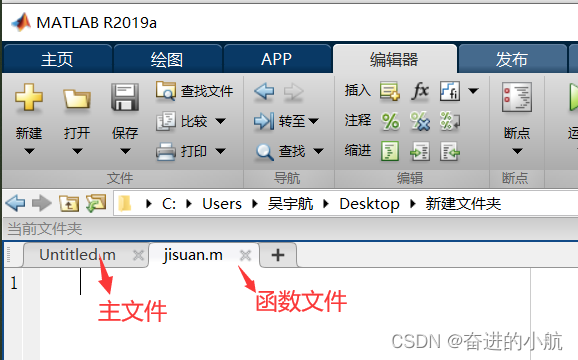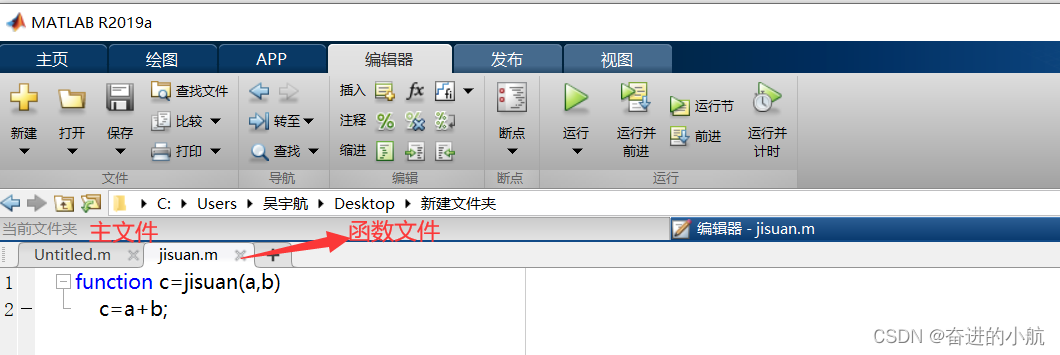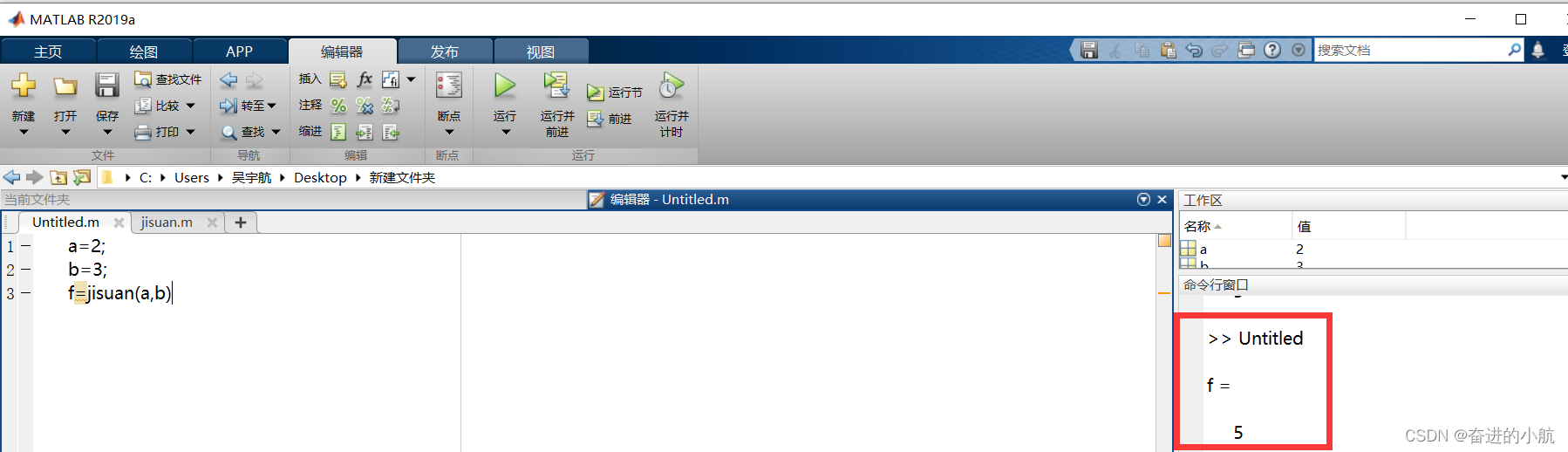• matlab创建函数并调用
万次阅读
2020-11-15 11:22:01

Matlab函数
函数是组织好的，可重复使用的，用来实现单一，或相关联功能的代码段。

函数能提高应用的模块性，和代码的重复利用率。你已经知道Matlab提供了许多内建函数，比如disp()。但你也可以自己创建函数，这被叫做用户自定义函数

matlab

disp(‘hello world’)
hello world
总的来说，自定义函数分为两步：即定义函数和调用函数。

定义一个函数
你可以定义一个由自己想要功能的函数，以下是简单的规则：

函数代码块以 function关键词开头，后接输出变量和函数标识符名称和圆括号 ()。
任何传入参数和自变量必须放在圆括号中间，圆括号之间可以用于定义参数。
函数的第一行语句可以选择性地使用文档字符串—用于存放函数说明。
函数内容以end结束。
函数需要定义在脚本文件（*.m）中运行，不可以在命令窗口中临时创建。

语法
Matlab定义函数需要使用function关键字，一般格式如下：

matlab
function 输出变量 = 函数名（输入变量）
函数体
end
这里的输入变量和输出变量都可以根据实际情况缺省。

实例
创建一个函数，用来输出“hello world!” ，步骤如下：

新建一个脚本，命名为eg1.m

matlab
test();
function test()
disp(‘hello world!’)
end
点击顶部菜单的运行按钮，即可在命令窗口输出：

matlab

eg1
hello world!
更复杂一点的应用，我们让函数带上输入变量和输出变量：

matlab
s = calc_area(4,5);
function s = calc_area(w,h)
s = w*h;
disp([‘area=’,num2str(s)]);
end
以上实例输出：

matlab

eg2
area=20
输入变量缺省
有时候，输入变量的个数不确定，但是又想将不同个数情况统一到一个函数里，即输入变量有缺省时，可以利用nargin来判断，然后给出对应的输出。例如：

matlab
out(1)
out(1,2)
out(1,2,3)
function out(a,b,c)
if nargin == 1
disp(a)
elseif nargin == 2
disp([a,b])
elseif nargin == 3
disp([a,b,c])
end
end
上面的实例将会得到：

matlab

eg5
1

 1     2

1     2     3


可以看到，当输入不同输入变量个数时，会找到对应个数下的执行代码，返回相应的内容。

当然这种做法也存在弊端，当变量个数太多时，要写很多个判断；这种情况下可以尝试inputParser,这里我就不多解释了，有兴趣可以了解下。

函数调用
函数基本结构完成后，进行调用时，存在两种方法：

直接在函数所在脚本文件内调用（上面的例子就是这种情况）；
函数定义与调用分开，各自存为一个脚本，运行调用脚本。
法一：脚本内调用函数
直接在函数脚本内调用
直接在函数脚本内调用

如上图所示，调用函数在前，定义函数在后，点运行即可得到输出。

法二：函数脚本独立，另外写代码调用
调用函数
调用函数

定义函数
定义函数

如上图所示，函数脚本和调用函数的脚本分开了，保存在同一路径下。

在第二种方法下，也可以在命令窗口直接调用所写的函数：

命令窗口调用函数
命令窗口调用函数

这种调用与上面本质上是一样的，都是首先找到以函数名命名的脚本文件，然后再执行函数内部代码块，返回结果。在这里必须注意函数脚本名字要与函数名一致。

事实上，为了让编程变得系统化，模块化，我们不建议你采用第一种方法去写代码，因为这样不利于分析和维护代码。

而采用后面一种方法时，我们可以定义很多个函数，然后在其他脚本里调用它们，这样形成了一种模块化的关系。我们在分析代码时，注意力可以集中到主程序上，思路将更加清晰。

输出值缺省调用
在matlab的函数中，可以选择性地获取所返回的变量列表值，下面给出实例：

matlab
default = out()
[a,,]= out()
[,b,] = out()
[,,c] = out()
function [a,b,c] = out()
a = 1;
b = 2;
c = 3;
end
上述实例输出结果：

matlab

eg4

default =

 1


a =

 1


b =

 2


c =

 3


可以看到，函数默认以返回的第一个变量为输出值；若想得到返回的某个值时，用变量接住，对于不需要的值，则需要用~占位，但不接收这个值，这样做的目的就是为了保证输出的序号能够匹配到。matlab
更多相关内容
• 首先是基本格式： function [ , , , ] = function_name ( , , , ) 方括号中的是输出变量，小括号中的是输入变量。 function 是固定格式，function_name 是你自己取得函数名称...编写好函数就是调用，在调用的过程中只需

首先是基本格式
function [ , , , ] = function_name ( , , , )

方括号中的是输出变量，小括号中的是输入变量。
function 是固定格式，function_name 是你自己取得函数名称。

值得注意的是，只有一个输出变量时，则不需要加方括号如果根本没有输出变量, 包括等号在内的都不需要了，如果没有输入变量, 函数名后面的括号可有可无。

尤其需要注意的是函数名和你的文件命必须一致

下面就是一个例子：编写好函数就是调用，在调用的过程中只需要调用函数名及具体参数即可。
示例如下：展开全文matlab
• matlab中如何自定义一个函数并进行调用

# 在matlab中如何自定义一个函数并进行调用？

## 定义一个加法函数并进行调用

### 1.创建一个主m文件，命名为任意名称，再创建一个名为函数名称的m文件，与主文件放在一个文件夹中，本例中函数名为jisuan### 2.在计算中自定义函数功能，固定的函数格式为：function result=函数名（参数1，参数2，…）。如图所示，该函数的功能是求参数a与参数b之和。其中c表示返回结果，在调用时可以任意命名，不影响最后结果，jisuan为函数名称，a为参数1，b为参数2，在调用时可以任意命名，不影响最后结果。### 3.在主文件里进行调用。定义变量a=2，定义变量b=3，想要求a和b之和f，需要调用函数f=jisuan（a,b），结果为5## summary：函数功能在调用时只要保证函数名称不变即可，剩余名称均可变动，保证位置对应即可

展开全文matlab
• matlab 定义函数,matlab定义函数并调用,Matlab自定义函数详解很久以前写的一篇Matlab自定义函数访问量很大，可惜没有点赞的，我感觉是我没讲清楚，这里又写了一篇笔记Matlab函数函数是组织好的，可重复使用的，用来...

matlab 定义函数,matlab定义函数并调用,Matlab自定义函数详解

很久以前写的一篇Matlab自定义函数访问量很大，可惜没有点赞的，我感觉是我没讲清楚，这里又写了一篇笔记

Matlab函数

函数是组织好的，可重复使用的，用来实现单一，或相关联功能的代码段。

函数能提高应用的模块性，和代码的重复利用率。你已经知道Matlab提供了许多内建函数，比如disp()。但你也可以自己创建函数，这被叫做用户自定义函数

disp('hello world')

hello world

总的来说，自定义函数分为两步：即定义函数和调用函数。

定义一个函数

你可以定义一个由自己想要功能的函数，以下是简单的规则：

函数代码块以 function关键词开头，后接输出变量和函数标识符名称和圆括号 ()。

任何传入参数和自变量必须放在圆括号中间，圆括号之间可以用于定义参数。

函数的第一行语句可以选择性地使用文档字符串—用于存放函数说明。

函数内容以end结束。

函数需要定义在脚本文件(*.m)中运行，不可以在命令窗口中临时创建。

Matlab定义函数需要使用function关键字，一般格式如下：

function 输出变量 = 函数名(输入变量)

这里的输入变量和输出变量都可以根据实际情况缺省。

创建一个函数，用来输出“hello world!” ，步骤如下：

新建一个脚本，命名为eg1.m

test();

function test()

disp('hello world!')

点击顶部菜单的运行按钮，即可在命令窗口输出：

eg1

hello world!

更复杂一点的应用，我们让函数带上输入变量和输出变量：

s = calc_area(4,5);

function s = calc_area(w,h)

s = w*h;

disp(['area=',num2str(s)]);

以上实例输出：

eg2

area=20

输入变量缺省

有时候，输入变量的个数不确定，但是又想将不同个数情况统一到一个函数里，即输入变量有缺省时，可以利用nargin来判断，然后给出对应的输出。例如：

out(1)

out(1,2)

out(1,2,3)

function out(a,b,c)

if nargin == 1

disp(a)

elseif nargin == 2

disp([a,b])

elseif nargin == 3

disp([a,b,c])

上面的实例将会得到：

eg5

1 2 3

可以看到，当输入不同输入变量个数时，会找到对应个数下的执行代码，返回相应的内容。

当然这种做法也存在弊端，当变量个数太多时，要写很多个判断；这种情况下可以尝试inputParser,这里我就不多解释了，有兴趣可以了解下。

函数基本结构完成后，进行调用时，存在两种方法：

直接在函数所在脚本文件内调用(上面的例子就是这种情况)；

函数定义与调用分开，各自存为一个脚本，运行调用脚本。

法一：脚本内调用函数如上图所示，调用函数在前，定义函数在后，点运行即可得到输出。

法二：函数脚本独立，另外写代码调用如上图所示，函数脚本和调用函数的脚本分开了，保存在同一路径下。

在第二种方法下，也可以在命令窗口直接调用所写的函数：这种调用与上面本质上是一样的，都是首先找到以函数名命名的脚本文件，然后再执行函数内部代码块，返回结果。在这里必须注意函数脚本名字要与函数名一致。

事实上，为了让编程变得系统化，模块化，我们不建议你采用第一种方法去写代码，因为这样不利于分析和维护代码。

而采用后面一种方法时，我们可以定义很多个函数，然后在其他脚本里调用它们，这样形成了一种模块化的关系。我们在分析代码时，注意力可以集中到主程序上，思路将更加清晰。

输出值缺省调用

在matlab的函数中，可以选择性地获取所返回的变量列表值，下面给出实例：

default = out()

[a,~,~]= out()

[~,b,~] = out()

[~,~,c] = out()

function [a,b,c] = out()

a = 1;

b = 2;

c = 3;

上述实例输出结果：

eg4

default =

展开全文• Matlab作为一款专业性极强的商业数学软件，将诸多的算法开发、统计分析、数据可视化功能融入其中，用户可以方便...再创建一个自定义函数test_data，将test_data函数文件放在当前文件夹内；在命令行窗口中输入简单...
• 此函数将创建一个树结构，其中包含作为输入给出的 m 文件中的所有函数调用。 该函数还将自动搜索被调用的 m 文件。 调用自身的函数不会创建无限循环。 您可以设置'搜索区域'，fi 该功能将只搜索'.\work\' 中的m 文件...matlab
• 目录一、建立文件二、函数调用二、函数句柄三、匿名函数想查找相关内容也可在页面按ctrl+F搜索文字一、建立文件新建脚本，保存后可运行，后缀为.m 二、函数调用创建ffib.m文件，编写求Fibonacci数列的函数function...
• Matlab作为一款专业性极强的商业数学软件，将诸多的算法开发、统计分析、数据可视化功能融入其中，用户可以方便...再创建一个自定义函数test_data，将test_data函数文件放在当前文件夹内；在命令行窗口中输入简单...
• 要实现的是调用Matlab函数，求矩阵前k个最小的特征值及其特征向量。 //C++ #include engine.h //使用Matlab引擎需要包含的头文件 #include using namespace std; int main() { Engine *m_engine; //创建Matlab...
• Matlab自定义函数的七种方法1、函数文件+调用命令文件：需单独定义一个自定义函数的M文件。2、函数文件+子函数：定义一个具有多个自定义函数的M文件。3、Inline:无需M文件，直接定义。4、匿名函数。5、Syms+subs:...
• 由伯克利大学附属的CSI于1975年创建的SAP2000软件是功能最强大的软件之一。 CSI的软件已被160多个国家的数千家工程公司用于主要项目的设计，其中包括台湾的台北101大楼，纽约世界贸易中心一号楼，北京的2008年...matlab
• 函数调用在一个m文件中，可以定义多个函数，但是文件名一定要与第一个函数(主函数)一致，该文件中其他函数则为本文件的私有函数，外部不可访问(可以通过参数调用的方法访问，下面会描述)。例如在一个程序中，创建一...
• 这是非法的，因为它将是调用此脚本的任何上下文中的脚本名称和变量名称。 出错 youhua100_50 (line 13) F1(m)=abs(sum(exp(sqrt(-1)*fai).*fBest')); 请问这个要怎么解决呢？这个是我在书上的原程序弄出来的，要...
• 创建一个用于探索自定义函数行为的滑块。 使用management.m 和“play-around”将您的函数输入附加到滑块，使用值查看函数的响应。 这对于交互式绘图特别有用。 帮助文字： 操纵（@（ARGS）FUNC（ARGS），{LABEL，...matlab
• matlab里怎么调用函数每一滴泪，每一道疤，是自己成长的代价，小编曾经犹豫过，葛然回首，小编不后悔。先保存你所编写的函数函数 function drawsur(surface), switch surface case 'sphere',sphere case '...
• 第1步：打开MATLAB首先，请通过按屏幕左下角的Windows按钮或按键盘上的Windows键来打开开始菜单。然后，在搜索栏中键入MATLAB，然后选择“MATLABR2013a”。请注意，如果您的MATLAB版本较旧，则程序的确切名称可能会...
• Python自带的函数有限，想要解决更多的问题，我们需要学会自己创建函数。 def（即define）：指定义一个函数 arg（即argument）：参数 return：返回结果 其中def和return是关键字，Python是靠识别这
• 使用格式良好的文档为 MATLAB 函数创建自定义模板。 可选参数包括（对于每个参数，可以在函数代码顶部轻松修改默认值）： - # 必要的参数- # 可选参数（即 VARARGIN）[解析为“名称”，值对 - 请参阅下文了解更多...matlab
• Matlab作为一款专业性极强的商业数学软件，将诸多的算法开发、统计分析、数据可视化功能融入其中，用户可以方便地调用需要的函数，建立数学模型，为了满足你工作的需要，还...再创建一个自定义函数test_data，将...
• 1.创建函数 一般自己写函数时要注意函数的结构，其中包括：函数名，输入值，输出值，以下图为例，function创建函数，‘JEntropy’为函数名，（需要注意保存的m文件名应与函数名一致），否则创建函数不成立，等号...matlab
• python调用matlab函数1、matlab中python外部接口（API）的安装2、matlab.egine模块3、从Python传递数值到Matlab 1、matlab中python外部接口（API）的安装 第一次使用Matlab需要先安装一下python的API。 路径为：…\...
• 在 2010 年伦敦举行的 Matlab 会议上，Joe Hicklin 评论说他有兴趣在 Matlab 函数中看到改进的调用错误报告，所以这是我尝试提供一些这种能力。 该函数采用： - 调用函数名称的字符串- 错误所在参数的整数- 具有成...matlab
• 本文演示如何创建指向局部函数的句柄。如果函数返回指向局部函数的句柄，则可以在主函数外部调用局部函数。此方法可让您在单个文件中包含多个可调用函数。 在您的工作文件夹下的 ellipseVals.m 文件中创建以下函数。...matlab
• Python 调用 MATLAB 1、创建python=3.5虚拟环境 conda create --name py37_matlabpython=3.7 漫长的等待 2、激活环境 conda activate py37_matlab 进入 E:\Program Files\MATLAB\R2021b\extern\engines\python ...matlab python
• c++调用matlab函数，踩了不少坑，终于搞定matlab
• 目录 从 C++ 调用MATLAB函数 ...使用matlab::engine::MATLABEngine类的feval和fevalAsync成员函数从 C++ 调用 MATLAB®函数。当您要将函数参数从 C++ 传递给 MATLAB 和将函数执行的结果返回给 C++ 时，请使用这些函...matlab
• 函数挪用在一个m文件中，可以界说多个函数，但是文件名必定要与第一个函数(主函数)一概，该文件中其他函数则为本文件的私有函数，外部不行访问(可以经历参数挪用的要领访问，下面会形容)。比如在一个步骤中，创建一...
• mkdotfile 创建函数依赖项的 GraphViz 点语言表示。 mGraphViz 将有向图绘制到图形上。 这些功能只是一个大型项目的一小部分，用于在MATLAB中实现类似于doxygen的代码文档系统，而该系统没有任何外部程序依赖性。 ...matlab...